# PSAT Math : How to find the diagonal of a cube

## Example Questions

### Example Question #1 : How To Find The Diagonal Of A Cube

If a cube is 3” on all sides, what is the length of the diagonal of the cube?

4√3

9

3√3

3√2

27

3√3

Explanation:

General formula for the diagonal of a cube if each side of the cube = s

Use Pythagorean Theorem to get the diagonal across the base:

s2 + s2 = h2

And again use Pythagorean Theorem to get cube’s diagonal, then solve for d:

h2 + s2 = d2

s2 + s2 + s2 = d2

3 * s2 = d2

d = √ (3 * s2) = s √3

So, if s = 3 then the answer is 3√3

### Example Question #1 : Solid Geometry

A cube is inscribed in a sphere of radius 1 such that all 8 vertices of the cube are on the surface of the sphere.  What is the length of the diagonal of the cube?

(3)

2

1

8

(2)

2

Explanation:

Since the diagonal of the cube is a line segment that goes through the center of the cube (and also the circumscribed sphere), it is clear that the diagonal of the cube is also the diameter of the sphere.  Since the radius = 1, the diameter = 2.

### Example Question #3 : How To Find The Diagonal Of A Cube

What is the length of the diagonal of a cube with volume of 512 in3?

2√(6) in

4√(3) in

8√(3) in

8 in

8√(3) in

Explanation:

The first thing necessary is to determine the dimensions of the cube.  This can be done using the volume formula for cubes: V = s3, where s is the length of the cube. For our data, this is:

s3 = 512, or (taking the cube root of both sides), s = 8.

The distance from corner to corner of the cube will be equal to the distance between (0,0,0) and (8,8,8).  The distance formula for three dimensions is very similar to that of 2 dimensions (and hence like the Pythagorean Theorem):

d√( (x1 – x2)2 + (y1 – y2)2  + (z1 – z2)2

Or for our simpler case:

d = √( (x)2 + (y)2  + (z)2) = √( (s)2 + (s)2  + (s)2) = √( (8)2 + (8)2  + (8)2) = √( 64 + 64 + 64) = √(64 * 3) = 8√(3)

### Example Question #4 : How To Find The Diagonal Of A Cube

What is the length of the diagonal of a cube with volume of 1728 in3?

3√(3) in

12√(3) in

18 in

12 in

6√(3) in

12√(3) in

Explanation:

The first thing necessary is to determine the dimensions of the cube.  This can be done using the volume formula for cubes: V = s3, where s is the length of the cube. For our data, this is:

s3 = 1728, or (taking the cube root of both sides), s = 12.

The distance from corner to corner of the cube will be equal to the distance between (0,0,0) and (12,12,12).  The distance formula for three dimensions is very similar to that of 2 dimensions (and hence like the Pythagorean theorem):

d = √( (x1 – x2)2 + (y1 – y2)2  + (z1 – z2)2

Or, for our simpler case:

d = √( (x)2 + (y)2  + (z)2) = √( (s)2 + (s)2  + (s)2) = √( (12)2 + (12)2  + (12)2) = √( 144 + 144 + 144) = √(3 * 144) = 12√(3) = 12√(3)

### Example Question #5 : How To Find The Diagonal Of A Cube

What is the length of the diagonal of a cube with surface area of 294 in2?

21√(2)

14

21

7√(3)

7√(3)

Explanation:

The first thing necessary is to determine the dimensions of the cube. This can be done using the surface area formula for cubes: A = 6s2, where s is the length of the cube. For our data, this is:

6s2 = 294

s2 = 49

(taking the square root of both sides) s = 7

The distance from corner to corner of the cube will be equal to the distance between (0,0,0) and (7,7,7). The distance formula for three dimensions is very similar to that of 2 dimensions (and hence like the Pythagorean Theorem):

d = √((x1 – x2)2 + (y1 – y2)2  + (z1 – z2)2

Or for our simpler case:

d = √((x)2 + (y)2  + (z)2) = √( (s)2 + (s)2  + (s)2) = √( (7)2 + (7)2  + (7)2) = √( 49 + 49 + 49) =  √(49 * 3) = 7√(3)

### Example Question #1 : How To Find The Diagonal Of A Cube

A rectangular prism has a volume of 144 and a surface area of 192. If the shortest edge is 3, what is the length of the longest diagonal through the prism?Explanation:

The volume of a rectangular prism is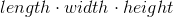.

We are told that the shortest edge is 3.  Let us call this the height.

We now have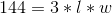, or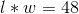.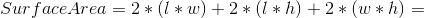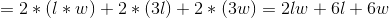Now we replace variables by known values: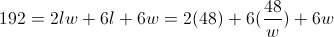Now we have: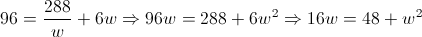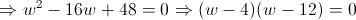We have thus determined that the other two edges of the rectangular prism will be 4 and 12.  We now need to find the longest diagonal.  This is equal to: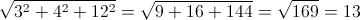If you do not remember how to find this directly, you can also do it in steps.  You first find the diagonal across one of the sides (in the plane), by using the Pythagorean Theorem.  For example, we choose the side with edges 3 and 4.  This diagonal will be: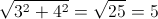We then use a plane with one side given by the diagonal we just found (length 5) and the other given by the distance of the 3rd edge (length 12).

This diagonal is then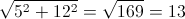.

### All PSAT Math Resources